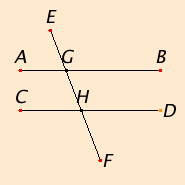# Proposition 28

If a straight line falling on two straight lines makes the exterior angle equal to the interior and opposite angle on the same side, or the sum of the interior angles on the same side equal to two right angles, then the straight lines are parallel to one another.

Let the straight line EF falling on the two straight lines AB and CD make the exterior angle EGB equal to the interior and opposite angle GHD, or the sum of the interior angles on the same side, namely BGH and GHD, equal to two right angles.I say that AB is parallel to CD.

Since the angle EGB equals the angle GHD, and the angle EGB equals the angle AGH, therefore the angle AGH equals the angle GHD. And they are alternate, therefore AB is parallel to CD.

Next, since the sum of the angles BGH and GHD equals two right angles, and the sum of the angles AGH and BGH also equals two right angles, therefore the sum of the angles AGH and BGH equals the sum of the angles BGH and GHD.

Subtract the angle BGH from each. Therefore the remaining angle AGH equals the remaining angle GHD. And they are alternate, therefore AB is parallel to CD.

Therefore if a straight line falling on two straight lines makes the exterior angle equal to the interior and opposite angle on the same side, or the sum of the interior angles on the same side equal to two right angles, then the straight lines are parallel to one another.

Q.E.D.

## Guide

This proposition states two useful minor variants of the previous proposition. The three statements differ only in their hypotheses which are easily seen to be equivalent with the help of proposition I.13.

#### Use of Proposition 28

This proposition is used in IV.7, VI.4, and a couple times in Book XI.